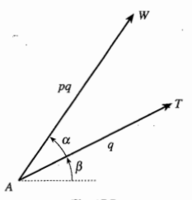Spiral enlargement

Dr.Peterson

Elite Member
I believe what they mean by "spiral enlargement" is that we obtain AW by multiplying AT by 2 and rotating it by pi/3. And that is just what they have done.

If that is not enough to help, please identify what specifically you are still confused by.

burgerandcheese

Junior Member
I believe what they mean by "spiral enlargement" is that we obtain AW by multiplying AT by 2 and rotating it by pi/3. And that is just what they have done.

If that is not enough to help, please identify what specifically you are still confused by.
I don't understand how they got 2(cos(pi/3) + i*sin(pi/3))Dr.Peterson

Elite Member
I don't understand how they got 2(cos(pi/3) + i*sin(pi/3))That is 2e^(pi/3 i), which is a multiplication by 2, and a rotation by pi/3 radians, which is just what they said they wanted to do.

If that is not clear, what do you know about rotation of complex numbers? We have to connect this to something you understand, and there are a couple ways you might have seen this.

burgerandcheese

Junior Member
That is 2e^(pi/3 i), which is a multiplication by 2, and a rotation by pi/3 radians, which is just what they said they wanted to do.

If that is not clear, what do you know about rotation of complex numbers? We have to connect this to something you understand, and there are a couple ways you might have seen this.
What I know is if we have t = q(cos(β) + isin(β)) and represent it as vector AT and s = p(cos(α) + isin(α)), then w = st represented as a vector AW is rotated from AT anticlockwise by α radians or rotated from the same horizontal axis by (α + β) radians and has magnitude pq:This will sound weird but I don't think I know what I don't know.....

Last edited:

Dr.Peterson

Elite Member
So you do know that multiplying vectors multiplies their magnitudes and adds their angles.

So multiplying a number z by 2(cos(pi/3) + i*sin(pi/3)) will double the magnitude, and rotate by pi/3 radians (that is, add pi/3 to the angle of z). That's exactly what they want to do, right?

•burgerandcheese

burgerandcheese

Junior Member
So you do know that multiplying vectors multiplies their magnitudes and adds their angles.

So multiplying a number z by 2(cos(pi/3) + i*sin(pi/3)) will double the magnitude, and rotate by pi/3 radians (that is, add pi/3 to the angle of z). That's exactly what they want to do, right?
I think I finally get it now. Thank you

Subhotosh Khan

Super Moderator
Staff member
What I know is if we have t = q(cos(β) + isin(β)) and represent it as vector AT and s = p(cos(α) + isin(α)), then w = st represented as a vector AW is rotated from AT anticlockwise by α radians or rotated from the same horizontal axis by (α + β) radians and has magnitude pq:

View attachment 12574

This will sound weird but I don't think I know what I don't know.....
I want to point out that AT and AW, as expressed by q*e and p*q*ei(α+ß) are not really vectors - those are phasors. One main difference between vectors and phasors is that:

vector division (inverse of multiplication) is not defined.